Concepts

Class 10
Chapter 12 Class 10 - Electricity

Ohm's Law gives a relationship between Potential Difference and Electric Current

As per Ohm's Law

Current flowing through a conductor is

directly proportional to

potential difference across its ends

Provided physical conditions like temperature remains the same

V ∝ I

Example

 Electric Current in Amperes Potential Difference In Volts 5 50 6 60 7 70 4 40 3 30

In this case, if we see

• If electric Current Increases, Potential Difference increases
• If electric Current Decreases, Potential Difference decreases

From above we can say

Potential Difference is directly proportional to Electric Current

V ∝ I

V = constant × I

V/I = constant

This constant is known as Resistance.

V = IR

## What is Meaning of Resistance

The Property of Conductor due to which it opposes the flow of current through it

This is because Resistance is inversely Proportional to Electric Current

As per Ohm's Law

Resistance is always constant for a given electric wire of a particular shape at a particular temperature

it is calculated as follows

Resistance = Potential Difference/Current

### What is SI Unit of Resistance

It is Measured in ohm

1 Ohm = 1 Volt/1 Ampere

Explanation

We know that

Resistance = Potential Difference/Current

Resistance is Measured in Ohm,Potential Difference in Volt and Current in Ampere

Hence our formula becomes

1 ohm = 1 Volt/1 Ampere

1 Ω = 1 V/1 A

## Calculating Resistance

It is the Ratio of Potential Difference to Electric Current

We know that,

V = IR
V/I = R

 Electric Current in Amperes (I) Potential Difference In Volts (V) Resistance R = V/I 5 50 10 6 60 10 7 70 10 4 40 10 3 30 10

Note -
Resistance is always constant and is 10 in the above case.

Note - Resistance is Inversely Proportional to Current

It means that if we increase Resistance,Electric Current Decreases

If we decrease Resistance,Electric Current Increases

Explanation

We know that

V = IR

V/R = I

I = V/R

Case 1

Suppose V = 100 V ,R = 20 Ω

I = V/R = 100/20 = 5 A

Case 2

Suppose we increase Resistance from 20Ω to 25Ω

I = V/R

I = 100/25 = 4A

Hence if Resistance increases ,Electric Current Decreases

Case 3

Similarly,if we decrease Resistance from 20Ω to 10Ω

I = V/R

I = 100/10 = 10A

Hence if Resistance Decreases, Electric Current Increases

This shows that Resistance is inversely Proportional to Electric Current

 Potential Difference V Resistance R Current I=V/R (V) (Ω) (A) 100 20 100/20 = 5 100 25 100/25 = 4 100 10 100/10 = 10

## Questions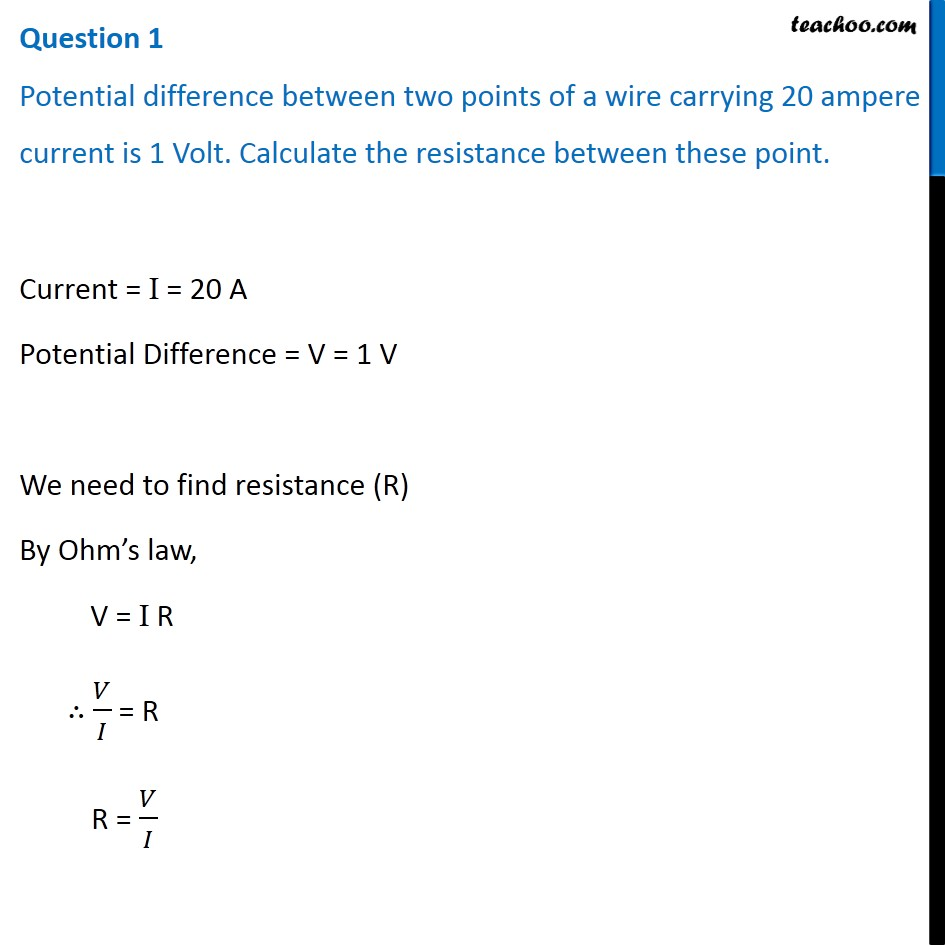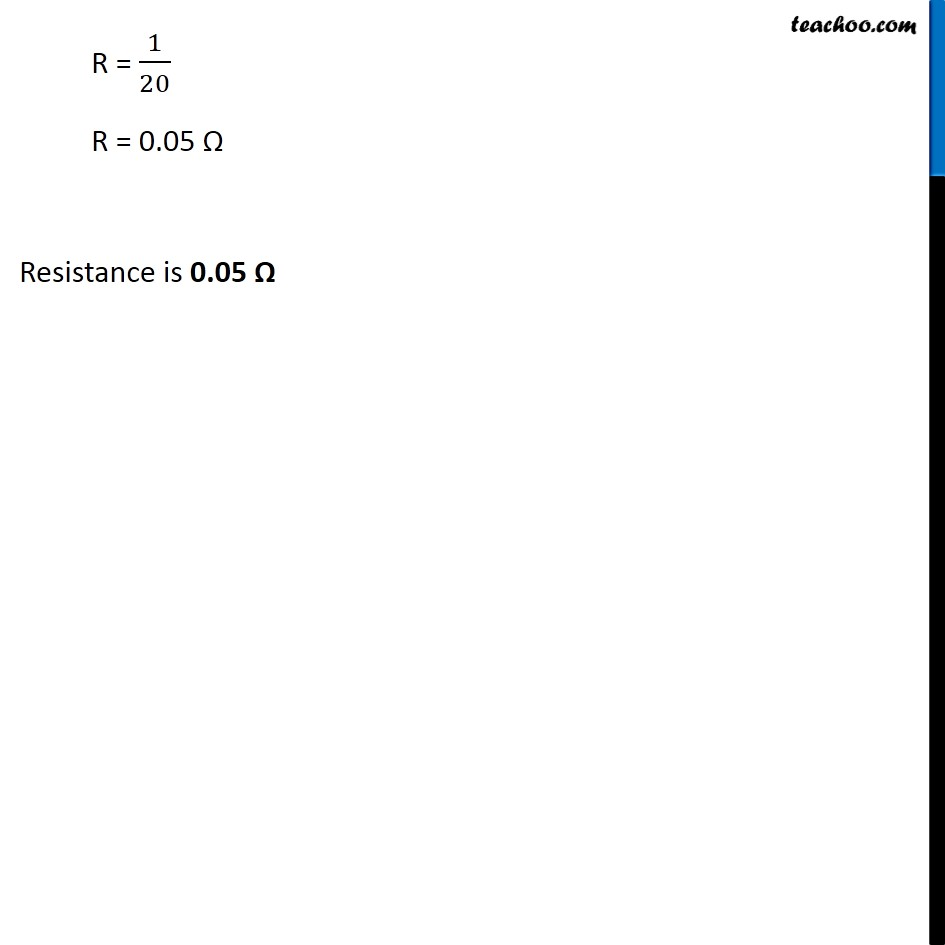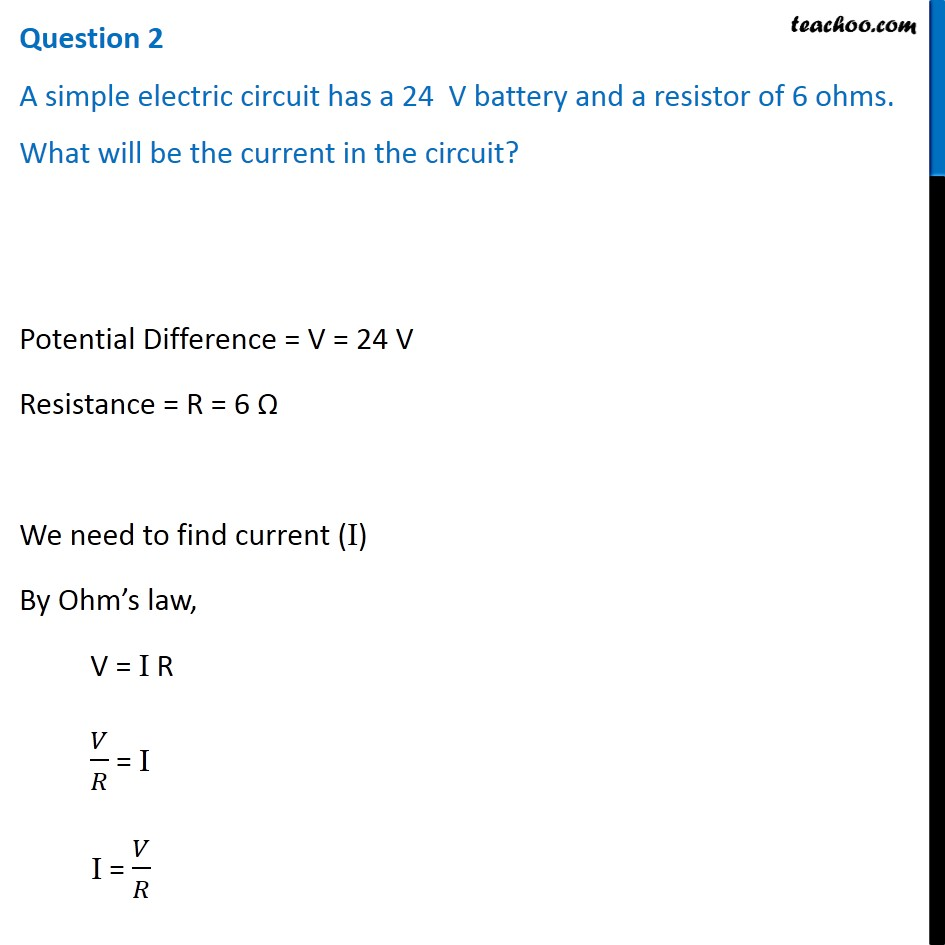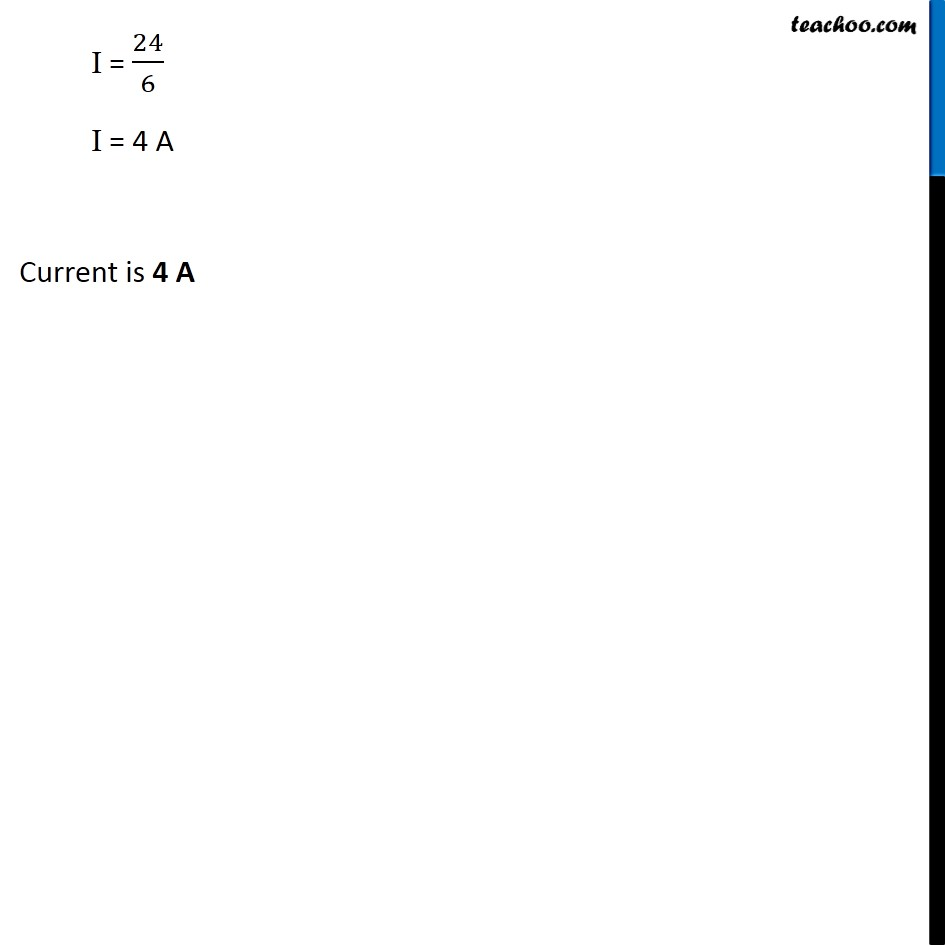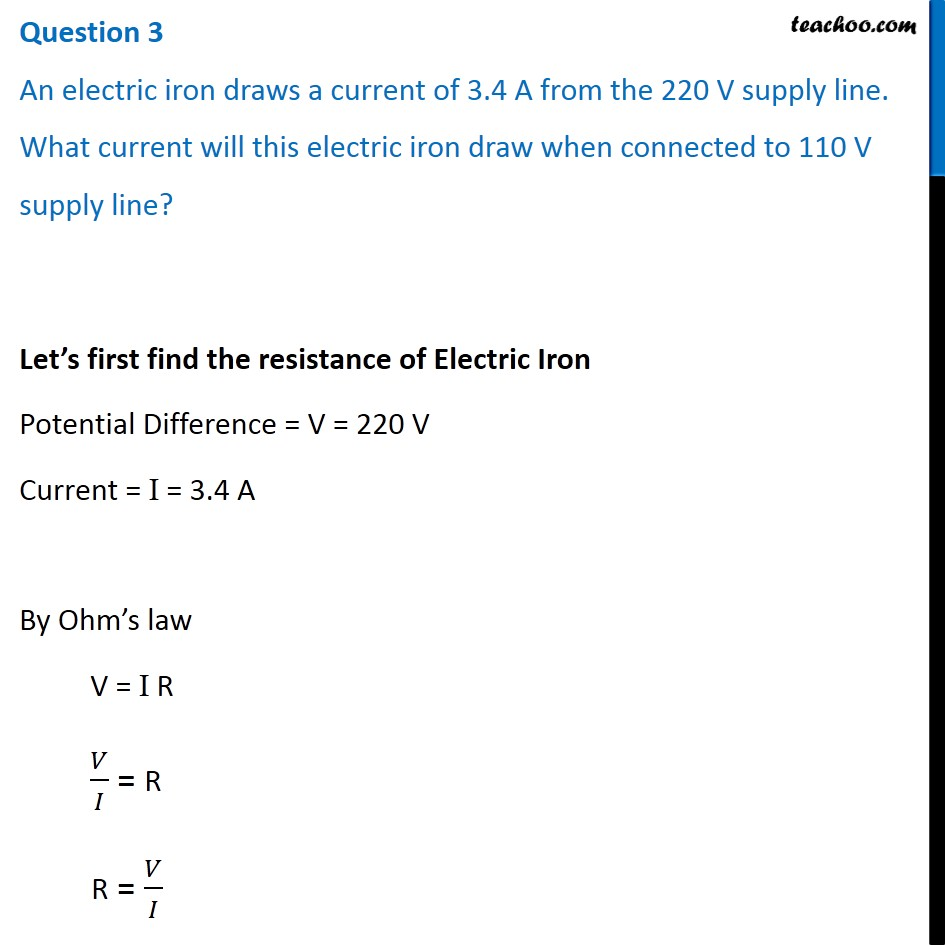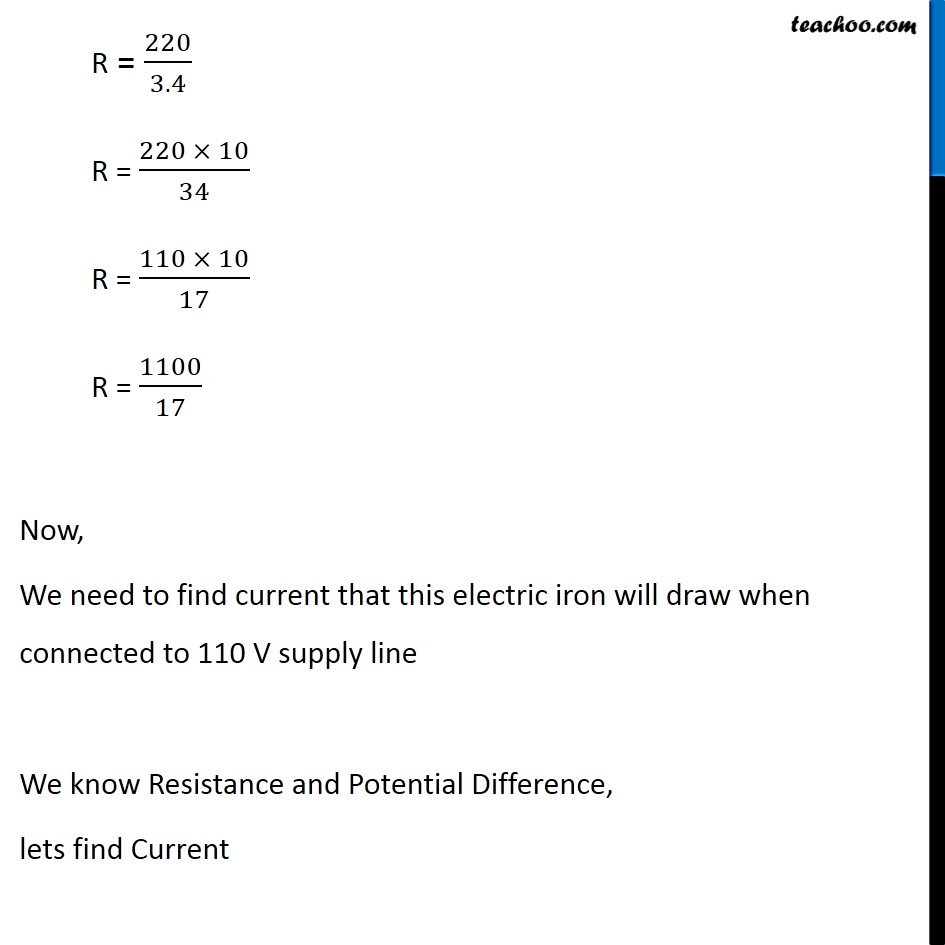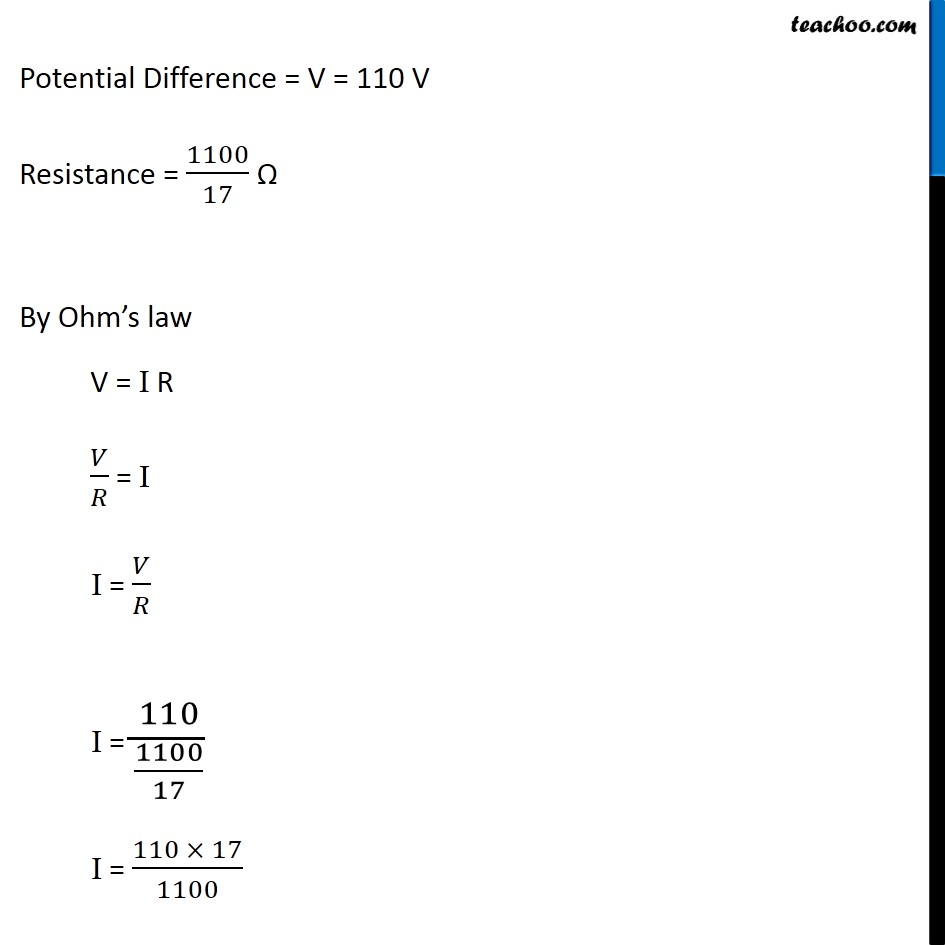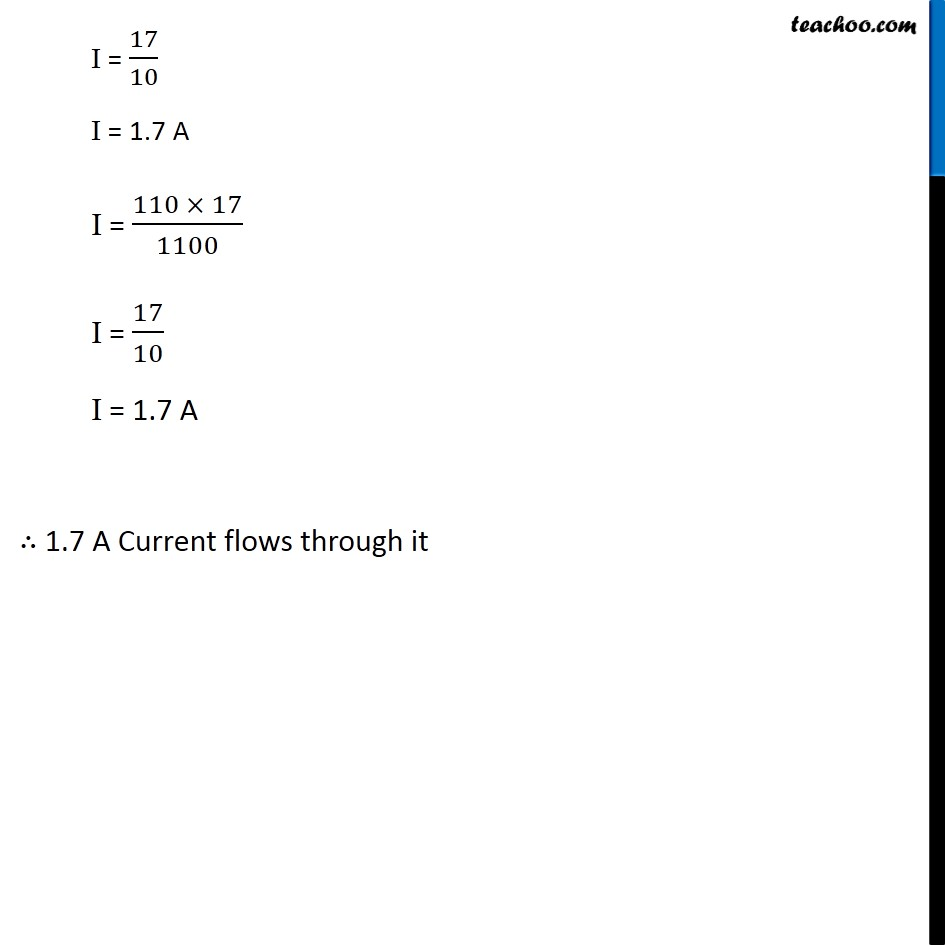## Questions

NCERT Question 7 - The values of current I flowing in a given resistor for the corresponding values of potential difference V across the resistor are given below –
I (amperes) 0.5 1.0 2.0 3.0 4.0
V (volts) 1.6 3.4 6.7 10.2 13.2
Plot a graph between V and I and calculate the resistance of that resistor.

NCERT Question 8 - The values of current I flowing in a given resistor for the corresponding values of potential difference V across the resistor are given below –
I (amperes) 0.5 1.0 2.0 3.0 4.0
V (volts) 1.6 3.4 6.7 10.2 13.2
Plot a graph between V and I and calculate the resistance of that resistor.

Example 12.3 - How much current will an electric bulb draw from a 220 V source, if the resistance of the bulb filament is 1200 Ω? (b) How much current will an electric heater coil draw from a 220 V source, if the resistance of the heater coil is 100 Ω?

Example 12.4 - The potential difference between the terminals of an electric heater is 60 V when it draws a current of 4 A from the source. What current will the heater draw if the potential difference is increased to 120 V?

Learn in your speed, with individual attention - Teachoo Maths 1-on-1 Class

### Transcript

Question 1 Potential difference between two points of a wire carrying 20 ampere current is 1 Volt. Calculate the resistance between these point. Current = I = 20 A Potential Difference = V = 1 V We need to find resistance (R) By Ohm’s law, V = I R ∴ 𝑉/𝐼 = R R = 𝑉/𝐼 R = 1/20 R = 0.05 Ω Resistance is 0.05 Ω Question 2 A simple electric circuit has a 24 V battery and a resistor of 6 ohms. What will be the current in the circuit? Potential Difference = V = 24 V Resistance = R = 6 Ω We need to find current (I) By Ohm’s law, V = I R 𝑉/𝑅 = I I = 𝑉/𝑅 I = 24/6 I = 4 A Current is 4 A Question 3 An electric iron draws a current of 3.4 A from the 220 V supply line. What current will this electric iron draw when connected to 110 V supply line? Let’s first find the resistance of Electric Iron Potential Difference = V = 220 V Current = I = 3.4 A By Ohm’s law V = I R 𝑉/𝐼 = R R = 𝑉/𝐼 R = 220/3.4 R = (220 × 10)/34 R = (110 × 10)/17 R = 1100/17 Ω Now, We need to find current will this electric iron draw when connected to 110 V supply line We know Resistance and Potential Difference, lets find Current Potential Difference = V = 110 V Resistance = 1100/17 Ω By Ohm’s law V = I R 𝑉/𝑅 = I I = 𝑉/𝑅 I = 110/(1100/17) I = (110 × 17)/1100 I = 17/10 I = 1.7 A I = (110 × 17)/1100 I = 17/10 I = 1.7 A ∴ 1.7 A Current flows through it